### Internal Forces: Shear and moment equations and their diagrams

The internal forces and couple moments in a loaded beam vary along a beam due to the loading conditions (e.g. number of external forces and their distances to the location of the internal forces) are different for different sections of the beam. Consequently, the values of the internal forces and couple moments along a beam must be known for a beam design. For example, maximum values of the shear force and bending moment and their locations along a beam are needed during a beam design.

The internal forces and couple moment acting at any point along the axis of a beam can be determined by the method of sections. In this regard, we need to define the section with a reference point, normally from the left end of the beam. Hence, the location of the internal forces of interest is defined by the distance from the left end of the beam to the point of interest denoted by(Fig. 7.14). Therefore, shear and bending moment at each point become functions of x (and the external forces), i.e.,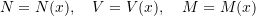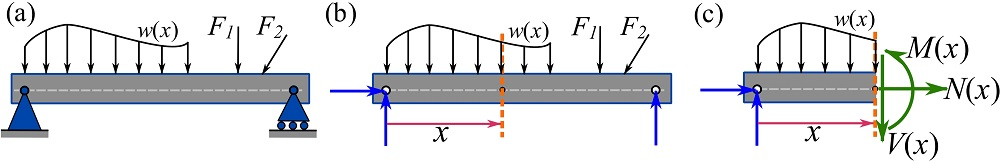Fig. 7.14 Sectioning a beam at an arbitrary point located away from the left end of a beam.

Remark: the signs of the results from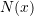,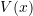, and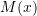refer to the member sign convention (Fig. 7.8, 7.9, and 7.10) because the unknown directions of internal forces are assumed positive according to that sign convention.

Structural beams are commonly long and straight members designed to carry loads mainly perpendicular to their long axes, upward or downward. Perpendicular loads on a beam affect only the shear force and bending moment within the beam, not the axial force In this case, axial force is zero anywhere along the beam.

The functionsandand their diagrams (graphs or plots) provide powerful tools for evaluating the values and variations of the shear force and bending moment at any point. The following example demonstrates how to obtainandusing the method of sections and plotting them. Theandplots are referred to as shear force diagram (SFD) and bending moment diagram (BMD) respectively.

### Example 7.2.1

Determine theandfor the loaded beam shown in the figure.

#### SOLUTION

1- Draw the FBD of the beam.

In the FBD, the directions of the unknown force and moment are assumed positive according to the member sign convention.

2- Solve the equations of equilibrium for the support reactions.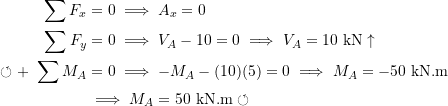3- Make a cut in the FBD of the beam at an arbitrary point x meter away from the left end of the beam as shown. Choose one of the two segments for analysis. For instance, the segment chosen is as shown below.

4- Write the equations of equilibrium for the resultant segment and solve for the shear force and bending moment at,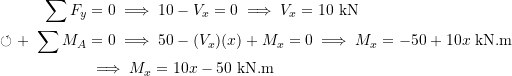Therefore,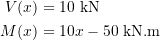5- Plot the functionsandon xy plots, with the x axis representing the distance from the left end of the beam, and the y axis representing the values ofand. Theplot gives a shear force diagram (SFD) and theplot gives a bending moment diagram (BMD).

In general, the loading condition on a section between the reference end and the cut will change as the cut moves from one end of the beam to the other, the inputs (external load) to theandwill change. Therefore,andmay need to be specifically defined for different sections  along the axis of a beam. A beam should be divided into sections along its axis (length) at locations where the external load pattern on the FBD of a beam changes. Figure 7.15 shows the sections needed for determiningand.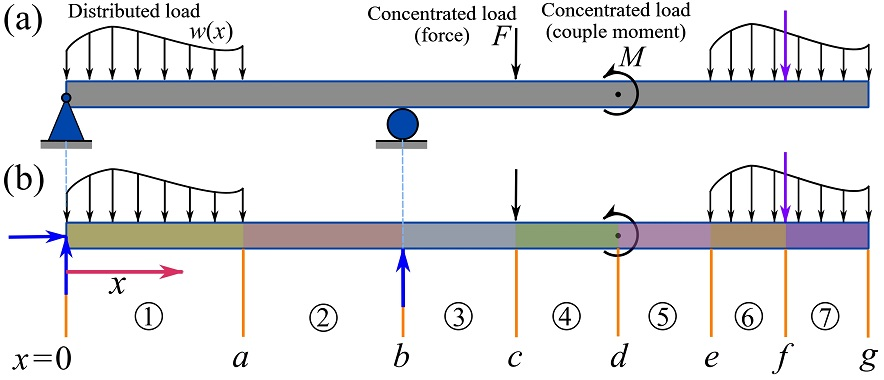Fig. 7.15 Locations (indicated by letters) at which the external load pattern changes and  the regions (indicated by numbers in circles) that different sets of and are needed.

### Example 7.2.2

Draw the shear force and bending moment diagrams of the loaded beam shown in the figure.

#### SOLUTION

1- Draw the FBD of the beam.

2- Solve the equations of equilibrium for the support reactions.3- Divide the beam (its FBD) into regions based on pattern change of the external loads.

4- In each region, cut at an arbitrary pointmeter away from the left end of the beam, write the equations of equilibrium, and solve forand.

Arbitrary cut in region 1.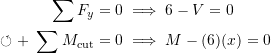The notation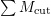means sum of the moments about the point at which the imaginary cut is made.

Therefore,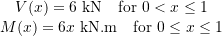Note that the region fordoes not include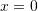. The reaction force atis a concentrated force under which the shear force has a rapid change (see Example 7.1.2) or mathematically a jump discontinuity. Therefore, the point at which a concentrated force acts should not be included in the region for.

Arbitrary cut in region 2.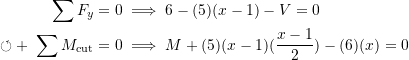Therefore,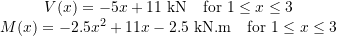Arbitrary cut in region 3.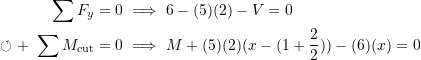Therefore,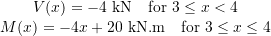Note that the region fordoes not include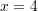. We will see that there will be a jump discontinuity in the diagram ofat. The shear force at the location of a concentrated force changes rapidly from one side of the concentrated force to the other side. We just need to know the shear forces on the two sides right next to the concentrated force.

Arbitrary cut in region 4.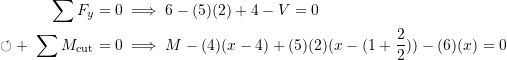Therefore,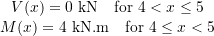Note that the region fordoes not include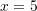. We will see that there will be a jump discontinuity in the diagram ofat. The bending moment at the location of a couple moment changes rapidly from one side of the couple moment to the other side. We just need to know the bending moments on the two sides right next to the couple moment.

Arbitrary cut in region 5.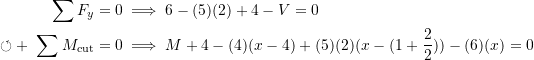Therefore,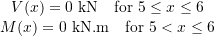5- Plot each functions for each region over the length of the beam.

Collecting the functions over all the regions, you can write,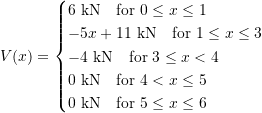and,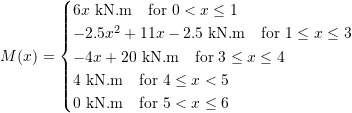The SFD and BMD are as follow.

Remark: Any point at which a concentrated force is applied is associated with a jump discontinuity in, this point should not be included in the region over whichis being determined. Similarly, Any point at which a couple moment is applied is associated with a jump discontinuity in, this point should not be included in the region over whichis being determined.

In the above example, we can visually notice the following effects and changes in the diagrams.

1- A point force creates a jump discontinuity in SFD.

2- A couple moment creates a jump discontinuity in BMD.

3- A discontinuity in the distributed load creates a sudden change of slope (i.e. discontinuity in the slope) in SFD.

4- A point force (also) creates a sudden change of slope in BMD.

These observations are not limited to this problem, but rather they follow general rules that are formally presented in the next section.